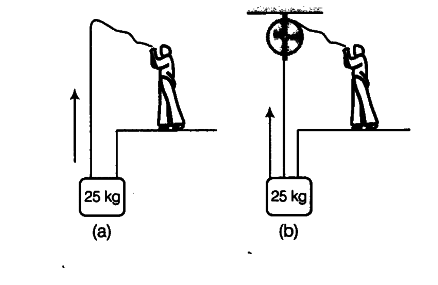# A block of mass 25 kg is raised by a 50 kg man in two different ways as shown in figure

A block of mass 25 kg is raised by a 50 kg man in two different ways as shown in figure. What is the action on the floor by the man in the two cases? If the floor yields a normal force of 700 N, which mode should the man adopt to lift the block without the floor yielding?Mass of block m = 25 kg
Mass of the man M = 50 kg
Force required to lift the block (F) = weight of the block,
F = mg = 25x10 = 250 N Weight of the man, w = Mg = 50 x 10 = 500 N Case (a)
If the block is raised by the man as shown in Fig. (a), then force is applied by the man in the upward direction due to which apparent weight of the man increases. Therefore action on the floor by the man = F + w
= 250 + 500= 750 N
Case (b)
If the block is raised by the man as shown in Fig. (b) then, force is applied by the man in the downward direction due to which apparent weight of the man decreases. Therefore, action on the floor by the man = mg — F
= 500 -250 = 250 N
The floor yields a normal force of 700 N. Action on the floor in case (a) exceeds 700 N, and less than 700 N in case (b). Therefore, mode (b) has to be adopted by the man to lift the block.